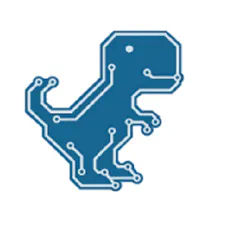# DIY Simple Frequency Meter Up to 6.5MHz

Another simple to build useful instrument in you lab.

BeginnerFull instructions provided15,699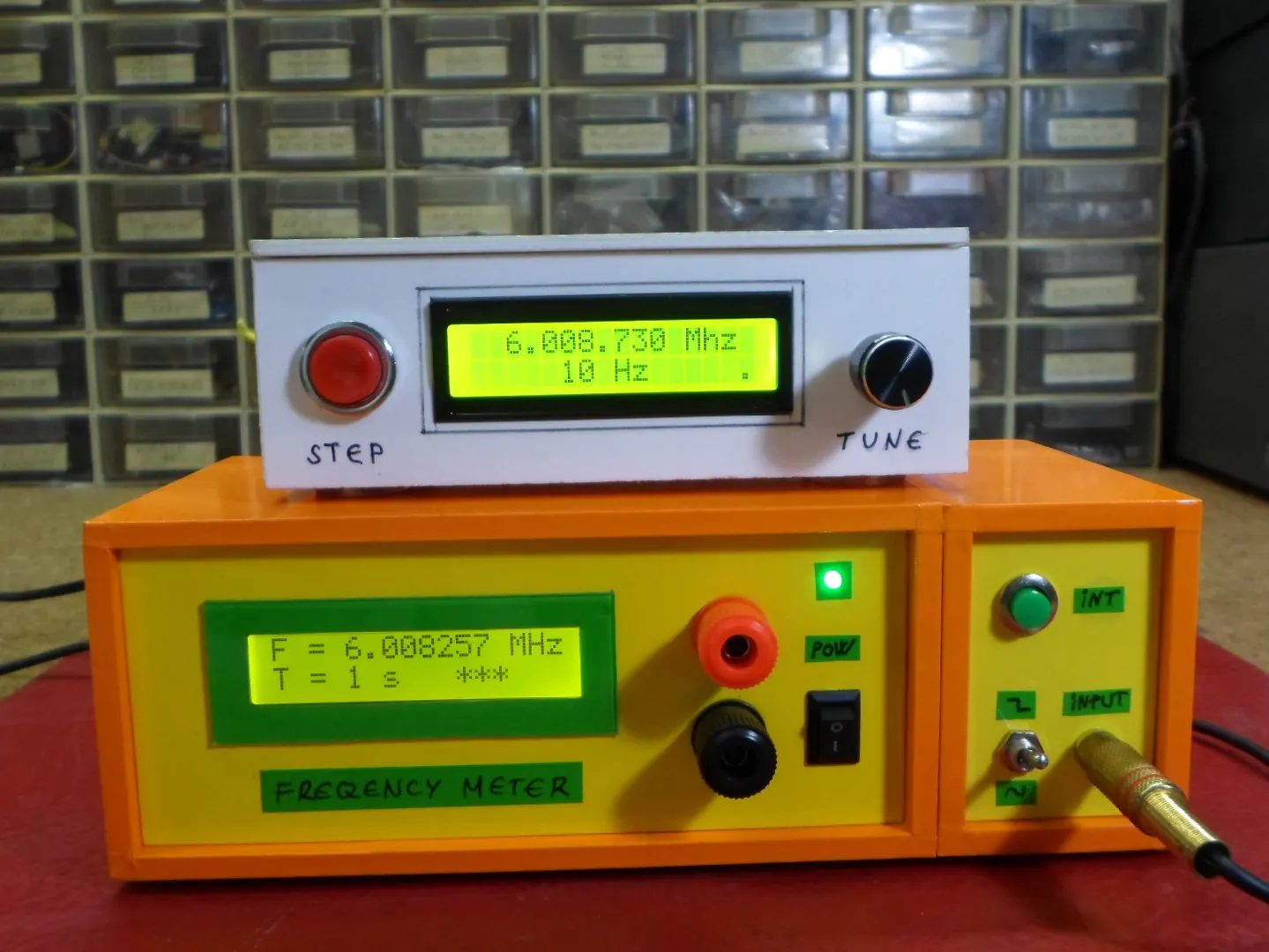## Things used in this project

### Hardware componentsArduino Nano R3
×1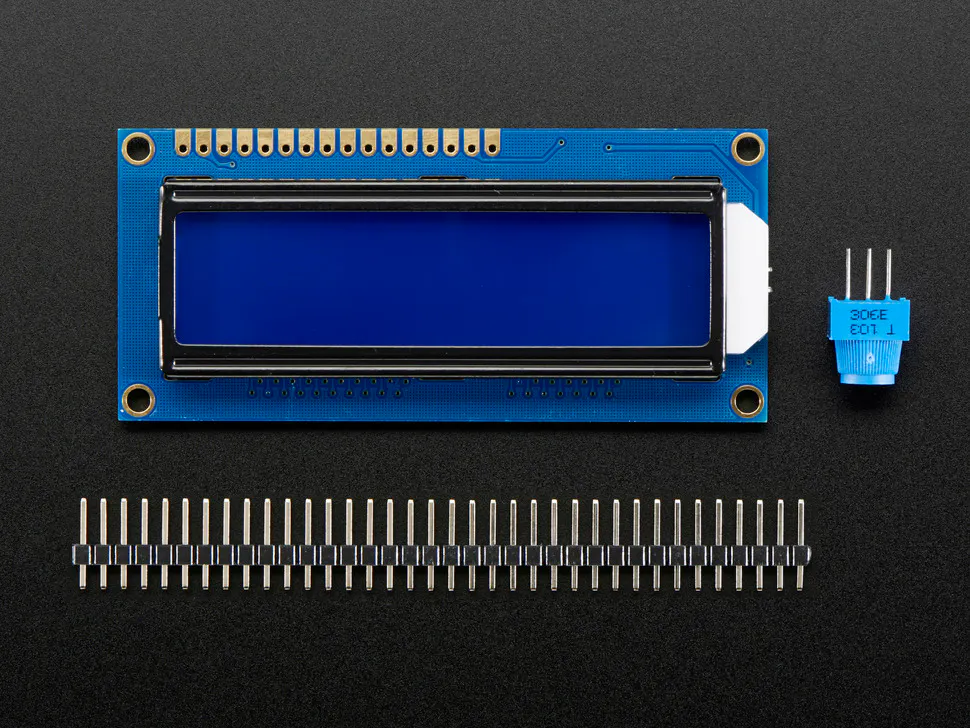Adafruit Standard LCD - 16x2 White on Blue
×1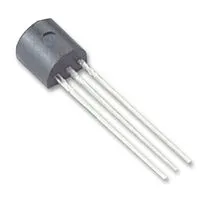General Purpose Transistor NPN
×1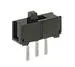Slide Switch
×1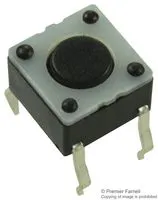Tactile Switch, Top Actuated
×1
 Resistors, capacitors, diodes
×1

### Software apps and online servicesArduino IDE

### Hand tools and fabrication machinesSoldering iron (generic)

## Schematics

### Schematic## Code

### Code

C/C++
```#include <FreqCount.h>//https://github.com/PaulStoffregen/FreqCount/archive/master.zip
#include <LiquidCrystal.h>

LiquidCrystal lcd(12, 11, 6, 4, 3, 2);// RS,E,D4,D5,D6,D7
void setup() {
lcd.begin(16, 2);// LCD 16X2
pinMode(7,INPUT);
FreqCount.begin(1000);
}
unsigned long f;float f0;
int x,n=3,r;

void loop() {

lcd.setCursor(0,1);
if(n==1){x++;if(x==1){FreqCount.begin(100);}r=-1;lcd.print("T = 0.1 s ");}
if(n==2){x++;if(x==1){FreqCount.begin(10000);}r=1;lcd.print("T = 10 s ");}
if(n==3){x++;if(x==1){FreqCount.begin(1000);}r=0;lcd.print("T = 1 s  ");}
if(n>3){n=1;}
lcd.setCursor(0,0);
lcd.print("F = ");
if(f>=1000000 && n==3){f0=f/1000000.0;lcd.print(f0,6+r);lcd.print(" MHz");}
if(f<1000000 && n==3){f0=f/1000.0;lcd.print(f0,3+r);lcd.print(" kHz");}
if(f>=100000 && n==1){f0=f/100000.0;lcd.print(f0,6+r);lcd.print(" MHz");}
if(f<100000 && n==1){f0=f/100.0;lcd.print(f0,3+r);lcd.print(" kHz");}
if(f>=10000000 && n==2){f0=f/10000000.0;lcd.print(f0,6+r);lcd.print("MHz");}
if(f<10000000 && n==2){f0=f/10000.0;lcd.print(f0,3+r);lcd.print(" kHz");}

if (FreqCount.available()) {

lcd.setCursor(10,1);lcd.print("***");
}
delay(200);
lcd.clear();
}
```

### FreqCount-master

C/C++
`No preview (download only).`

## Credits

### Mirko Pavleski

20 projects • 524 followers